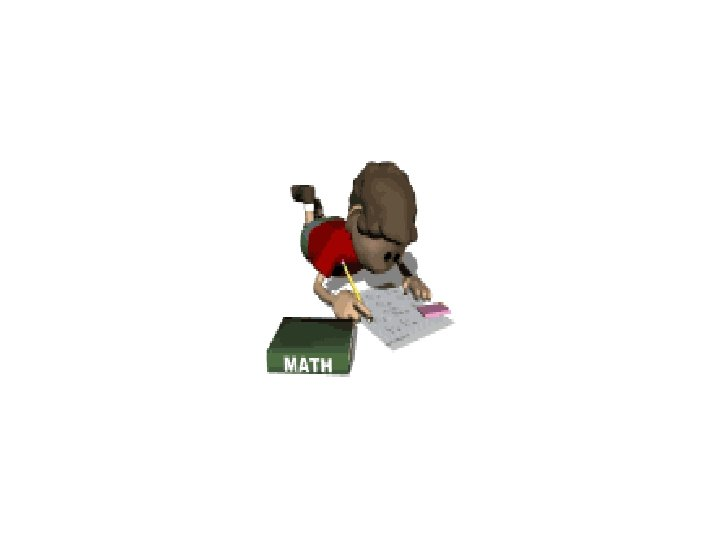# The US Customary System of Measurement AND CONVERSIONS

• Slides: 25
Download presentationThe US Customary System of Measurement AND CONVERSIONS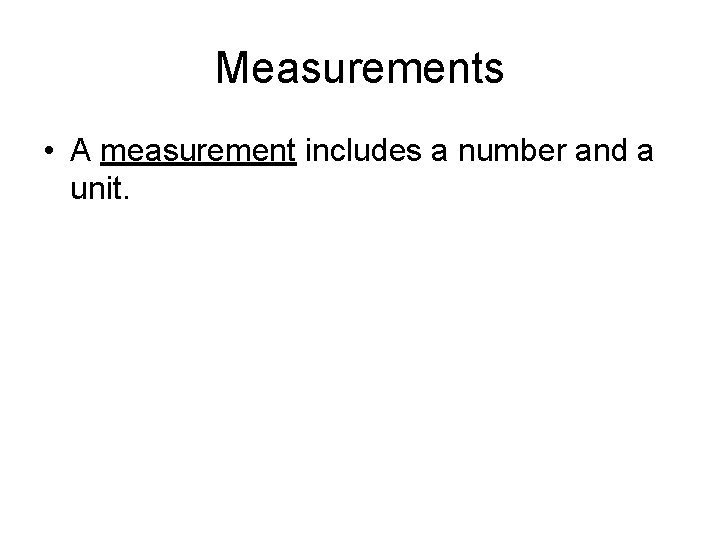Measurements • A measurement includes a number and a unit.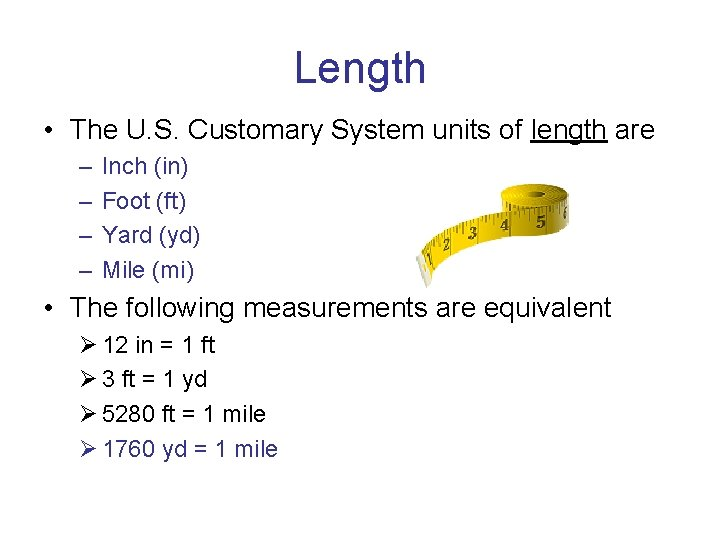Length • The U. S. Customary System units of length are – – Inch (in) Foot (ft) Yard (yd) Mile (mi) • The following measurements are equivalent Ø 12 in = 1 ft Ø 3 ft = 1 yd Ø 5280 ft = 1 mile Ø 1760 yd = 1 mileWeight • Weight is a measure of how strongly gravity is pulling on an object (decreases as elevation increases) • The U. S. Customary System units of weight are – Ounce (oz) – Pound (lb) – ton • The following measurements are equivalent: Ø 16 oz = 1 lb Ø 2000 lb = 1 tonCapacity • Liquid substances are measured in units of capacity. • The U. S. Customary System units of capacity are – – – Fluid ounces (fl oz) Cups (C) Pints (pt) Quarts (qt) Gallons (gal) • The following measurements are equivalent: Ø Ø 8 fl oz = 1 C 2 C = 1 pt 2 pt = 1 qt 4 qt = 1 galLand of gallonGallon Man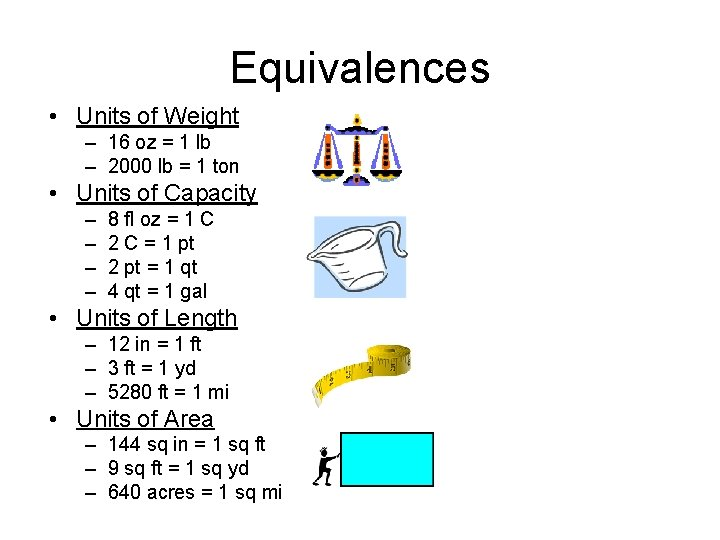Equivalences • Units of Weight – 16 oz = 1 lb – 2000 lb = 1 ton • Units of Capacity – – 8 fl oz = 1 C 2 C = 1 pt 2 pt = 1 qt 4 qt = 1 gal • Units of Length – 12 in = 1 ft – 3 ft = 1 yd – 5280 ft = 1 mi • Units of Area – 144 sq in = 1 sq ft – 9 sq ft = 1 sq yd – 640 acres = 1 sq mi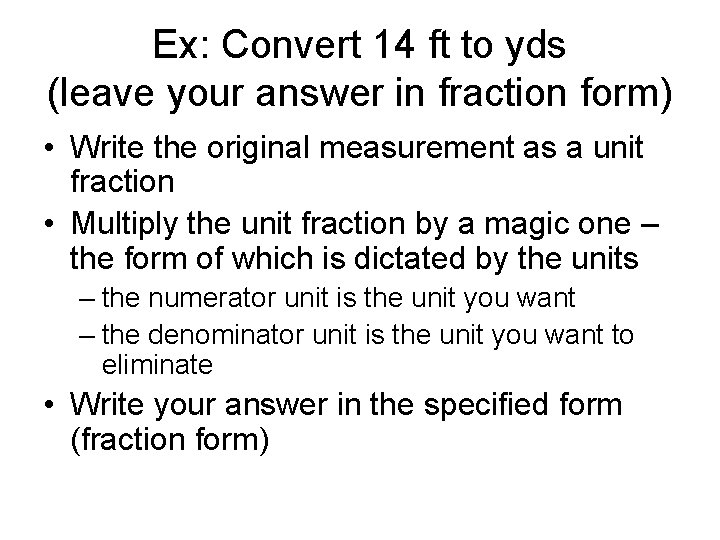Ex: Convert 14 ft to yds (leave your answer in fraction form) • Write the original measurement as a unit fraction • Multiply the unit fraction by a magic one – the form of which is dictated by the units – the numerator unit is the unit you want – the denominator unit is the unit you want to eliminate • Write your answer in the specified form (fraction form)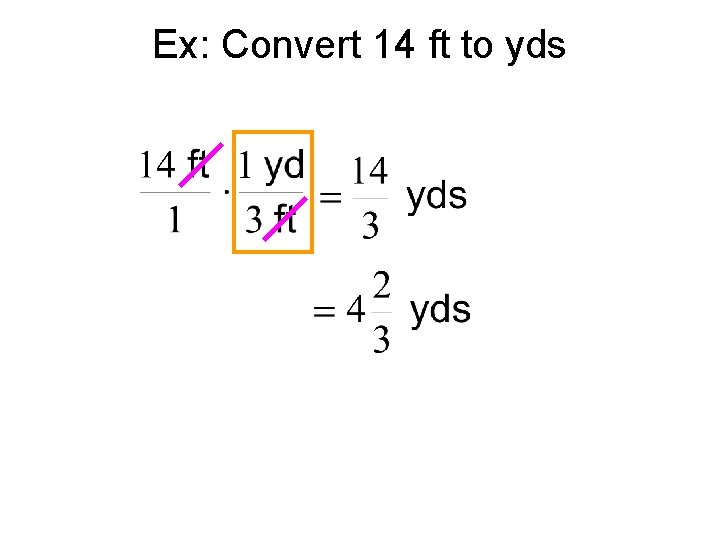Ex: Convert 14 ft to yds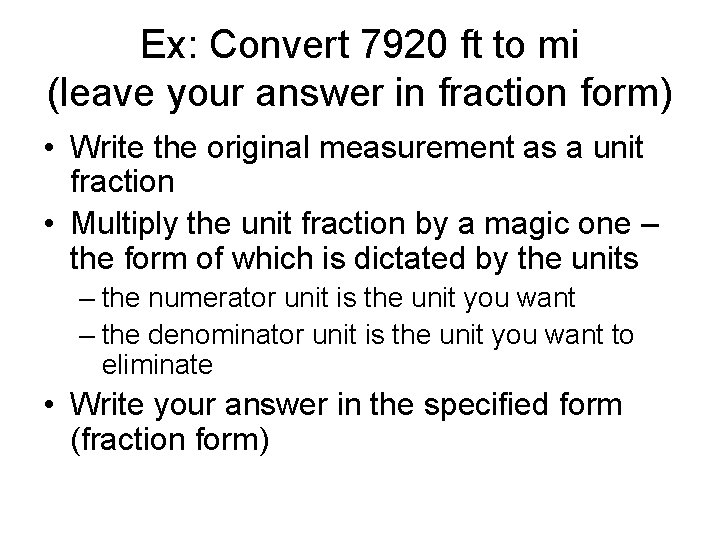Ex: Convert 7920 ft to mi (leave your answer in fraction form) • Write the original measurement as a unit fraction • Multiply the unit fraction by a magic one – the form of which is dictated by the units – the numerator unit is the unit you want – the denominator unit is the unit you want to eliminate • Write your answer in the specified form (fraction form)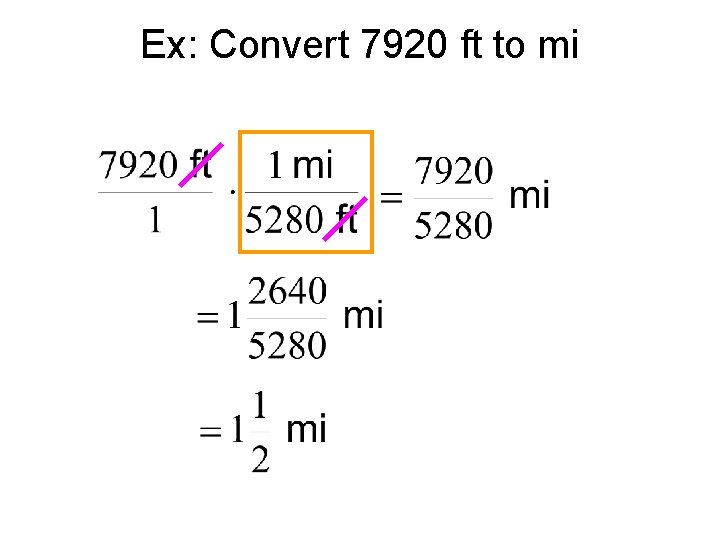Ex: Convert 7920 ft to mi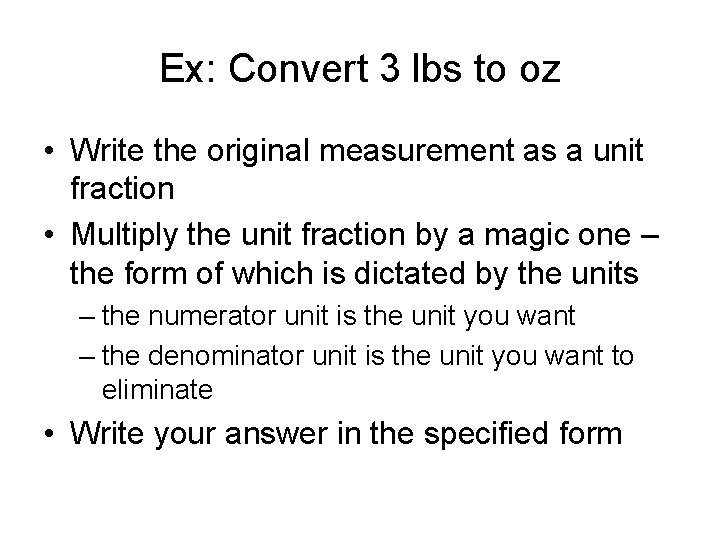Ex: Convert 3 lbs to oz • Write the original measurement as a unit fraction • Multiply the unit fraction by a magic one – the form of which is dictated by the units – the numerator unit is the unit you want – the denominator unit is the unit you want to eliminate • Write your answer in the specified formEx: Convert 3 lbs to oz = 48 ozEx: Convert 2 ¼ gal to qt • Write the original measurement as a unit fraction • Multiply the unit fraction by a magic one – the form of which is dictated by the units – the numerator unit is the unit you want – the denominator unit is the unit you want to eliminate • Write your answer in the specified formEx: Convert 2 ¼ gal to qt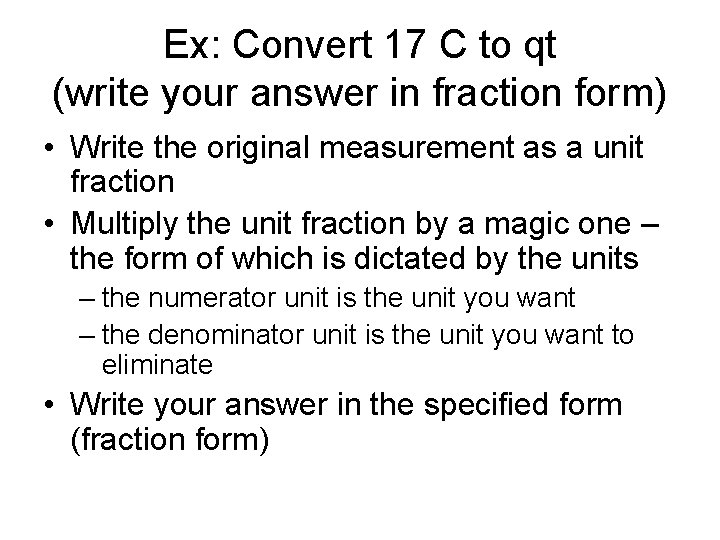Ex: Convert 17 C to qt (write your answer in fraction form) • Write the original measurement as a unit fraction • Multiply the unit fraction by a magic one – the form of which is dictated by the units – the numerator unit is the unit you want – the denominator unit is the unit you want to eliminate • Write your answer in the specified form (fraction form)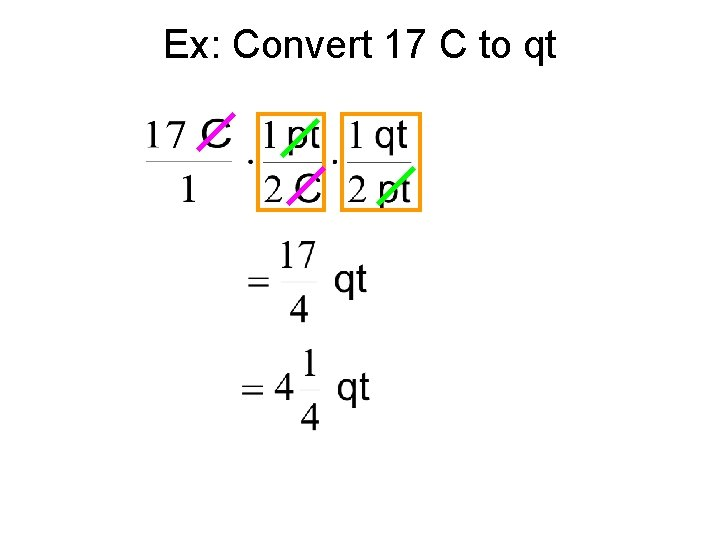Ex: Convert 17 C to qtEx: At a nursing staff meeting, 60 nurses are expected to attend. Each nurse is expected to drink 2 C of coffee. The industrial size coffee maker brews coffee in gallons. How many gallons of coffee should be prepared for the staff meeting? • How many cups of coffee is needed? • What should we do?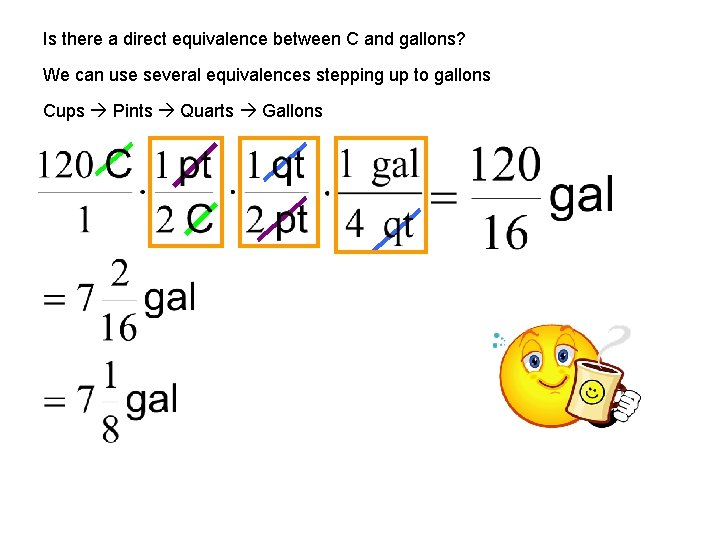Is there a direct equivalence between C and gallons? We can use several equivalences stepping up to gallons Cups Pints Quarts GallonsMore Practice Convert 25 yds to feet • Write the original measurement as a unit fraction • Multiply the unit fraction by a magic one – the form of which is dictated by the units – the numerator unit is the unit you want – the denominator unit is the unit you want to eliminate • Write your answer in the specified formEx: Convert 25 yds to feet = 75 ftEven More Practice Ex: Convert 60 in into ft • Write the original measurement as a unit fraction • Multiply the unit fraction by a magic one – the form of which is dictated by the units – the numerator unit is the unit you want – the denominator unit is the unit you want to eliminate • Write your answer in the specified formEx: Convert 60 in into ft = 5 ft